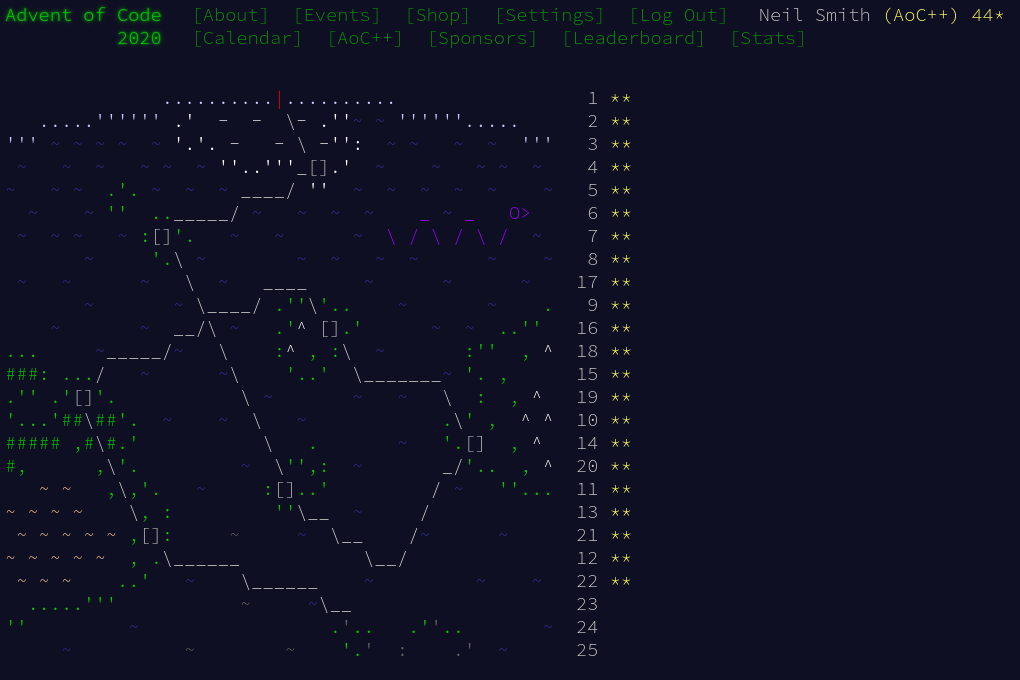January 6, 2021

# Advent of Code 2020 day 22

A simple game, with a DIY hash table for speedDay 22 was a chance to show off the Sequence library, a fast and easy-to-use implementation of double-ended queues.

I start by parsing the input into a pair of Sequences of cards.

type Deck = Q.Seq Int
type Game = (Deck, Deck)

decksP = (,) <$> deckP <* (many endOfLine) <*> deckP headerP = string "Player " *> decimal *> ":" *> endOfLine deckP = Q.fromList <$> (headerP *> (decimal sepBy endOfLine))


## Part 1

A single round of the game is mostly done for me with pattern matching and guards (the surrounding code ensures that the two sequences are non-empty). Similarly, detecting if the game is finished is all done with pattern matching.

finished :: Game -> Bool
finished (Empty, _)     = True
finished (_,     Empty) = True
finished (_,     _)     = False

playRound :: Game -> Game
playRound ((x :<| xs), (y :<| ys))
| x < y     = (xs,           ys |> y |> x)
| otherwise = (xs |> x |> y, ys)


I put those together with the standard until function (found with Hoogle) to play the game.

play = until finished playRound


That's it! There's a bit of post-processing of a game to extract the winning score.

part1 game = score $winningDeck$ play game

winningDeck :: Game -> Deck
winningDeck (Empty, ys) = ys
winningDeck (xs,    _)  = xs

score :: Deck -> Int
score = sum . zipWith (*) [1..] . toList . Q.reverse


## Part 2

I found the explanation of the recursive game a bit confusing, especially the behaviour around detecting repeated states. Imagine a sequence of games where there's a main game, which calls a recursive sub-game A; after that returns, sub-game B starts.

• When I'm checking for duplicates in sub-game B, should I consider the states encountered in sub-game A? (I decided "no.")
• When I find a duplicate in sub-game B, should I terminate just sub-game B or the main game as well? (I decided "just the sub-game.")

But with that, the implementation of the recursive game end up being a fairly mechanical translation from the description. Each game terminates with a notification of the winner and the winner's deck.

1. If either deck is empty, the other player wins
2. If the game is the same as a previous state, player 1 wins
3. If both players have enough cards, play a recursive round to find the winner, then play with the updated decks
4. Otherwise, compare top cards to find the winner, then play with the updated decks.
data Player = P1 | P2 deriving (Show, Eq)
type Cache = S.Set Game

playRecursive :: Game -> Cache -> (Player, Deck)
playRecursive (Empty, ys) _ = (P2, ys)
playRecursive (xs, Empty) _ = (P1, xs)
playRecursive g@(x :<| xs, y :<| ys) seen
| g S.member seen = (P1, x :<| xs)
| (lengthAtLeast x xs) && (lengthAtLeast y ys) = playRecursive subG seen'
| otherwise = playRecursive compareG seen'
where seen' = S.insert g seen
(subWinner, _) = playRecursive (Q.take x xs, Q.take y ys) seen'
subG = updateDecks subWinner g
compareTops = if x < y then P2 else P1
compareG = updateDecks compareTops g

updateDecks P1 (x :<| xs, y :<| ys) = (xs |> x |> y, ys)
updateDecks P2 (x :<| xs, y :<| ys) = (xs, ys |> y |> x)

lengthAtLeast n s = Q.length s >= n


That worked, but execution took a bit longer than I wanted. Profiling the runtime showed the program spending about 50% of its time inserting new games into the cache. I would leave things there, but Justin Le has a neat solution using hashes which I thought I'd try.

The idea is to have a hash table as the cache and generate a hash from the game state. The hash should be quick to generate and quick to test for membership. The hash table is a Map from hash values to Sets of game states. If the hash is not in the cache, we can't have seen this state before; we should add both the hash and the singleton set of this game. If the hash is in the cache, we can check the associated set for the definitive answer; if it's not there, we should add the game to the set.

playRecursive :: Game -> Cache -> (Player, Deck)
playRecursive (Empty, ys) _ = (P2, ys)
playRecursive (xs, Empty) _ = (P1, xs)
playRecursive g@(x :<| xs, y :<| ys) seen
| g inCache seen = (P1, x :<| xs)            -- < change here
| (lengthAtLeast x xs) && (lengthAtLeast y ys) = playRecursive subG seen'
| otherwise = playRecursive compareG seen'
where seen' = enCache g seen                   -- < change here
(subWinner, _) = playRecursive (Q.take x xs, Q.take y ys) seen'
subG = updateDecks subWinner g
compareTops = if x < y then P2 else P1
compareG = updateDecks compareTops g

hashGame (xs, ys) =
hash ( toList $Q.take 2 xs , toList$ Q.take 2 ys
)

inCache :: Game -> Cache -> Bool
inCache game cache = case (M.lookup h cache) of
Just games -> game S.member games
Nothing -> False
where h = hashGame game

enCache :: Game -> Cache -> Cache
enCache game cache = case (M.lookup h cache) of
Just games -> M.insert h (S.insert game games) cache
Nothing -> M.insert h (S.singleton game) cache
where h = hashGame game


That brings the runtime down to about 1.8 seconds.

## Code

You can find the code here or on GitLab.